Deutsche VersionSound pressure  p and the inverse distance law 1/r
for point-shaped sound sources in a free field (direct field)

How does the sound pressure (SPL) or the amplitude decrease with distance from the sound source?
How does the
sound pressure level decrease with doubling the distance from the source?
How can you calculate the distance drop of the sound?

 Avoid using the psychoacoustical terms loudness perception and volume. This subjective sound-sensation is not clearly measurable without ambiguity. The term "loudness" or "volume" is a problem because it belongs to psycho- acoustics and this personal feeling is not correct definable. Loudness as a psychological correlate of physical strength (amplitude) is also affected by parameters other than sound pressure, including frequency, bandwidth, spectral composition, information content, time structure, and the duration of exposure of the sound signal. The same sound will not create the same loudness perception by all individuals (people).

 As psycho-acoustic parameters to describe the "loudness" there is the "loudness level" with the unit phon and the "loudness" with the unit sone.

Sound intensity I is not sound pressure p.      I ~ p2 is true for progressive plane waves.

Compare also the inverse square law 1/r2,
when using sound intensity as sound energy quantity.
How does the sound decrease with distance?

This is an approximation when the venue is a direct sound field or an anechoic room

 In the real world, the inverse distance law p ~ 1/r is always an idealization because it assumes exactly equal sound pressure p as sound field propagation in all directions. If there are reflective surfaces in the sound field, then reflected sounds will add to the directed sound and you will get more sound at a field location than the inverse distance law predicts. If there are barriers between the source and the point of measurement, you may get less than the distance law predicts. Nevertheless, the inverse distance law is the logical first estimate of thesound pressure you would get at a distant point in a reasonably open area. The reference sound pressure level SPL = 0 dB is the sound pressure of p0 = 20 µPa = 20 × 10−6 Pa = 2 × 10−5 Pa or 2 × 10−5 N/m2. Field quantities, as the sound pressure, will always be shown as effective values (RMS).

 If you measure at distance  r1   = m = ft  a sound pressure level (SPL1)  Lp1 = dB,  and then at distance  r2   = m = ft  the distance law 1/r predicts a sound   pressure level (SPL2) of  Lp2 = dB in free field.
 You can explore numerically to confirm the 1/r law that doubling the distance drops the sound pressure p to a half (0.5) by a sound pressure level of about 6 dB and that 10 times the distance drops the sound pressure p to a tenth (0.1), that is a level drop by 20 dB.

 There is no noise decrease or sound drop per meter. We get a sound level drop of 6 dB per doubling of distance. Sound power or sound power level has nothing to do with the distance from the sound source.Note: The radiated sound power (sound intensity) is the cause and the sound pressure is the effect, where the sound engineer is particularly interested in the effect. The effect of temperature and sound pressure: Sound pressure and Sound power – Effect and Cause.

 Acousticians and sound protectors ("noise fighters") need the sound intensity (acoustic intensity) – but sound engineers and sound designers ("ear people") don't need that sound energy quantity.   Who is involved in audio engineering, should rather take care of the sound field quantity, that is the sound pressure or the sound pressure level (SPL) as an effect at the eardrums of our hearing and on the diaphragms of the microphones, and the corresponding audio voltage and its voltage level.

Sound pressure and Sound power − Effect and Cause

Sound pressure level and Sound pressure

 Enter a value in the left or right box. The calculator works in both directions of the ↔ sign.
 Sound pressure level Lp  dB-SPL ↔ Sound pressure p   Pa = N/m2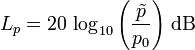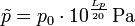Standard reference sound pressure p0 = 20 μPa or 2 × 10-5 Pa ≡ 0 dB

Sound waves move our eardrums (tympanic membranes).
But which sound quantity produces this effect?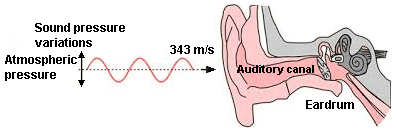Sound pressure and Sound power – Effect and Cause   Sound power is the cause - but sound pressure produces the audible effect.

Inverse distance law 1/r for sound pressure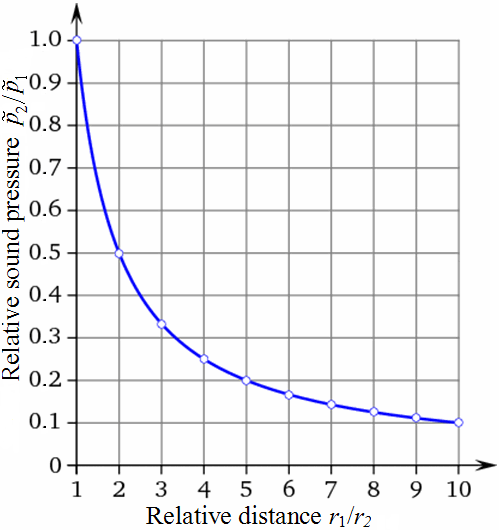Law for sound field quantities The graphs shown are normalized Distance ratio Sound pressure p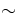1/r 1 1/1 = 1.0000 2 1/2 = 0.5000 3 1/3 = 0.3333 4 1/4 = 0.2500 5 1/5 = 0.2000 6 1/6 = 0.1667 7 1/7 = 0.1429 8 1/8 = 0.1250 9 1/9 = 0.1111 10 1/10 = 0.1000

Calculating sound pressure with the inverse distance law

 Relationship of sound pressure p, intensity I, and the distance law: (r is the distance from the sound source)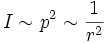This obviously means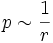Formulas for distance attenuation − Sound pressure calculation

 The RMS value of the sound pressure ͠p increases inversely with increasing distance from the sound source, that means with 1/r: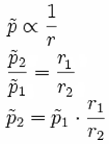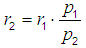Where: p1 = sound pressure 1 at closer distance r1 from the sound source p2 = sound pressure 2 at more far distance r2 from the sound source r1 = closer distance r1 from the sound source r2 = more far distance r2 from the sound source

 The sound pressure level Lp to plot against the distance of the sound source r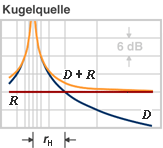D: direct field of the spheric source R: reflected field (diffuse field) rH: critical distance

 If we double the distance, the value for the sound pressure falls to a half (50%) of its initial value. If we double the distance, the value for the sound intensity falls to a quarter (25%) of its initial value. This corresponds to a decrease in level by (−)6 dB. For the level change in dB we get:How is the sound level dependent from the distance to the sound source?  The sound pressure level shows in the free field situation a reduction of 6 dB per  doubling of distance; that means the sound pressure drops to a half and not a quarter.  It is the sound intensity, that drops to a quarter of the initial value.

 A doubling of distance from the sound source in the direct field will reduce the "sound level" by (−)6 dB, no matter whether that are sound pressure levels or sound intensity levels! This will reduce the sound pressure p (field quantity) to 1/2 (50 %) and the sound intensity I (energy quantity) to 1/22 = 1/4 (25 %) of the initial value. The inverse distance law 1/r shows the distance performance of field quantities and the inverse square law 1/r2 shows the distance performance of energy quantities. Squared field quantities are proportional to energy quantities; e.g. p2 ~ I

 Damping of sound level in decibels with distance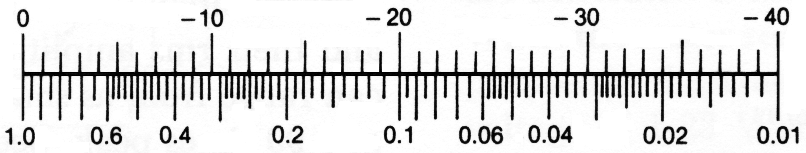Distance ratio

 Sound Field QuantitiesSound pressure, sound or particle velocity, particle displacement or displacement amplitude, (voltage, current, electric resistance). Inverse Distance Law 1/r Sound Energy Quantities Sound intensity, sound energy density, sound energy, acoustic  power. (electrical power). Inverse Square Law 1/r²

Frequently used false statements in the context of
sound values and the distance of the sound source

 Correct version Wrong expression Sound pressure (amplitude) falls inversely proportional to the distance 1/r from the sound source. That is the 1/r law or the inverse distance law. Sound pressure (amplitude) falls inversely proportional to the square of the distance 1/r2 from the sound source.                           Really wrong Sound pressure level decreases by (−)6 dB for doubling of the distance from the source to 1/2 (50 %) of the sound pressure initial value. Sound pressure level decreases inversely as the the distance increases for doubling of distance from the source by (−)3 dB.                             wrong Sound intensity (energy) falls inversely proportional to the square of the distance 1/r2 from the sound source. That is the inverse square law 1/r2. Sound intensity (energy) falls inversely proportional to the distance 1/r from the sound source.                                                    wrong Sound intensity level decreases by (−)6 dB for doubling of the distance from the source to 1/4 (25 %) of the sound intensity initial value. Sound intensity level decreases inversely as the square of the distance increases for doubling of distance from the source by (−)3 dB.         wrong

Sound pressure is not intensity

 Neither the sound power nor the sound power level decreases in doubling the distance. Why is this so? The sound power level quantifies the totally radiated sound energy from an object. Different to the sound pressure the sound power is independent of the distance to the sound source, the surrounding area and other influences.

 Differentiate: Sound pressure p is a "sound field quantity" and sound intensity I is a "sound energy quantity". In teachings these terms are not often separated sharply enough and sometimes are even set equal. But I ~ p2.

Changing of sound power with distance is nonsense

 Question: How does the sound power decrease with distance"? Answer: "April fool - The sound power does not decrease (drop) with distance from the sound source."   Levels of sound pressure and levels of sound intensity decrease equally with the distance from the sound source. Sound power or sound power level has nothing (!) to do with the distance from the sound source. Thinking helps: A 100 watt light bulb has in 1 m and in 10 m distance really always the same 100 watts, which is emitted from the lamp all the time. Watts don't change with distance.   A frequent question: "Does the sound power depend on distance?" The clear answer is: "No, not really."   We consider sound fields in air which are described by the scalar quantity p (sound pressure) and the vector quantity v (sound velocity) as a sound field quantity.

How does the sound level depend on distance from the source?

 Consider a source of sound and imagine a sphere with radius r, centered on the source. The sound source outputs a total power P, continuously. The sound intensity I is the same everywhere on this surface of a thought sphere, by definition. The intensity I is defined as the power P per unit area A. The surface area of the sphere is A = 4 π r2, so the sound intensity passing through each square meter of surface is, by definition: I = P / 4 π r2. We see that sound intensity is inversely proportional to the square of the distance away from the source: I2 / I1 = r12 / r22. But sound intensity is proportional to the square of the sound pressure, I ~ p2, so we can write: p2 / p 1 = r1 / r2. The sound pressure p changes with1 / r of the distance. So, if we double the distance, we reduce the sound pressure by a ratio of 2 and the sound intensity by a ratio of 4: in other words, we reduce the sound level by 6 dB. If we increase r by a ratio of 10, we decrease the level by 20 dB.

How many decibels (dB) level change is twice (double, half) or four times as loud?

 The beginners question is quite simply: How does the sound decrease with distance? More specifically questioned: How does the volume (loudness perception) decrease with distance? How does the sound pressure decrease with distance? How does the sound intensity (not the sound power) decrease with distance?

The decrease of sound with distance

 For a spherical wave we get: The sound pressure level (SPL) decreases with doubling of distance by (−)6 dB. The sound pressure falls to 1/2 times (50%) of the initial value of the sound pressure. The sound pressure drops with the ratio 1/r of the distance.   The sound intensity level decreases with doubling of distance also by (−)6 dB. The intensity falls to 1/4 times (25%) of the initial value of the sound intensity. The sound intensity (acoustic intensity) drops with the ratio 1/r2 of the distance.   For a cylindrical wave of a line source we get: The sound pressure level (SPL) decreases with doubling of distance only by (−)3 dB. The sound pressure falls to 0.707 times (70.7%) of the initial value of the sound pressure. The sound pressure drops with the ratio 1/√r to the distance. *) A loudspeaker line array operates according to this principle, but takes account of the finite length of the array. Therefore the sound pressure level (SPL) (!) of the low frequencies decrease (−)6 dB spherically with doubling of the distance.   The sound intensity level decreases with doubling of distance also by (−)3 dB. The intensity falls to 1/2 times (50%) of the initial value of the sound intensity. The sound intensity drops with the ratio 1/r to the distance. The acoustic power level does not decrease with the doubling of distance. A lamp with the power of 100 watts has in 1 m or in 10 m distance still the same watts.

 Note: The radiated sound power (sound intensity) is the cause - and the sound pressure is the effect. The effect is of particular interest to the sound engineer.

 Acousticians and sound protectors (noise fighters) need the sound intensity (acoustic intensity). As a sound designer you don't need that sound energy quantity. The eardrums of our hearing and the diaphragms of the microphones are effectively moved by the sound pressure or the sound pressure level.   Who is involved in audio engineering, should care less about the cause of intensity, power and energy, but better care on the effect of the sound pressure and the sound level (sound pressure level SPL) on the eardrums and the microphones, and the corresponding audio voltage or its voltage level.

Sound pressure and sound power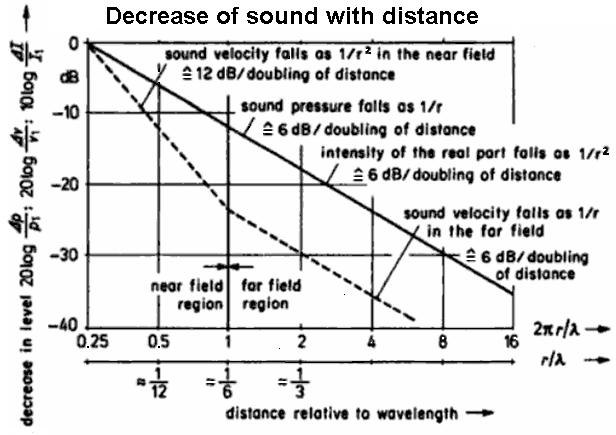Pressure, velocity, and intensity of the sound field near to and distant from a spherical radiator of the zeroth order

 "Ear people" like sound engineers and sound designers are mainly interested in sound field quantities and consider more the sound pressure drop at distance doubling (Schalldruckabfall - Entfernungsverdopplung). Acousticians and noise fighters are mainly interested in sound energy quantities and consider here the sound intensity drop at distance doubling. They all view together the same line!Isn't that beautiful? Nevertheless, the drop in sound pressure goes with 1/r and the decrease of sound intensity is 1/r2. This should be understood quite well.

 Our hearing (ear drum) is directly sensitive to the sound pressure. From the historical perspective, the level differences for stereo listening were called "intensity" differences. However, sound intensity is a specifically defined quantity that can not be picked up by a microphone, nor would it be useful for a sound recording. So call the "intensity" stereophony better level difference stereophony.   If you have to work as a technician to check the sound quality by ear, then think of the sound waves, which move the eardrums using the the sound pressure as a sound field quantity. There is also the advice: Avoid the use of sound power and sound intensity as sound energy quantities.

The law of distance or the distance law

The inverse square law 1/r² and the inverse distance law 1/r

 The radiation of light or the radiation of the radioactivity or the signal strength of a transmitting antenna (mobile) is inversely proportional to the square of the distance, thus assumes the square of the distance from (1/r²). So the sound intensity decreases as 1/r² from the square of the distance. This means here energy quantities or power quantities. "The longing for home increases with the square of the distance." ... but the sound pressure decreases just as 1/r as the distance of r - without any square. Sound pressure is, however, a field quantity.

 In audio, electronics and acoustics use only the word "damping" and not the wrong word "dampening".